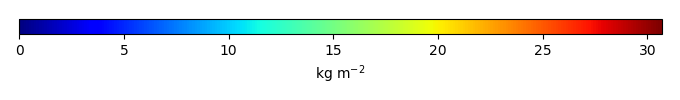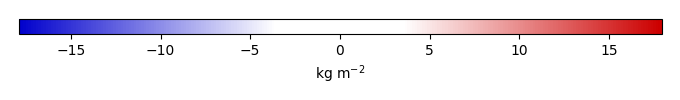# Mean State

Period Mean (original grids) [Pg]
Model Period Mean (intersection) [Pg]
Model Period Mean (complement) [Pg]
Benchmark Period Mean (intersection) [Pg]
Benchmark Period Mean (complement) [Pg]
Bias [kg m-2]
Bias Score 
Spatial Distribution Score 
Overall Score 
Benchmark [-] 30.1
CLM4 [-] 467. 18.2 448. 29.9 0.195 -1.10 0.59 0.59 0.59
CLM4.5 [-] 453. 21.1 432. 29.9 0.195 -0.690 0.58 0.72 0.65
CLM5 [-] 487. 22.3 465. 29.9 0.195 -0.481 0.58 0.77 0.67
Period Mean (original grids) [Pg]
Model Period Mean (intersection) [Pg]
Model Period Mean (complement) [Pg]
Benchmark Period Mean (intersection) [Pg]
Benchmark Period Mean (complement) [Pg]
Bias [kg m-2]
Bias Score 
Spatial Distribution Score 
Overall Score 
Benchmark [-] 1.44
CLM4 [-] 3.07 0.712 2.34 1.41 0.0279 -0.483 0.52 0.48 0.50
CLM4.5 [-] 7.93 2.85 4.96 1.41 0.0279 1.42 0.54 0.87 0.71
CLM5 [-] 14.5 5.95 8.42 1.41 0.0279 4.18 0.21 0.47 0.34
Period Mean (original grids) [Pg]
Model Period Mean (intersection) [Pg]
Model Period Mean (complement) [Pg]
Benchmark Period Mean (intersection) [Pg]
Benchmark Period Mean (complement) [Pg]
Bias [kg m-2]
Bias Score 
Spatial Distribution Score 
Overall Score 
Benchmark [-] 0.00649
CLM4 [-] 4.65 0.0130 4.37 0.00649 0.00 0.0455 0.42 0.091 0.26
CLM4.5 [-] 8.49 0.0210 8.21 0.00649 0.00 0.191 0.60 0.79 0.70
CLM5 [-] 10.2 0.0250 9.91 0.00649 0.00 0.233 0.58 0.75 0.67
Period Mean (original grids) [Pg]
Model Period Mean (intersection) [Pg]
Model Period Mean (complement) [Pg]
Benchmark Period Mean (intersection) [Pg]
Benchmark Period Mean (complement) [Pg]
Bias [kg m-2]
Bias Score 
Spatial Distribution Score 
Overall Score 
Benchmark [-] 17.4
CLM4 [-] 13.4 13.0 0.213 17.3 0.0180 -0.783 0.66 0.82 0.74
CLM4.5 [-] 14.7 14.2 0.283 17.3 0.0180 -0.425 0.66 0.91 0.78
CLM5 [-] 12.0 11.6 0.244 17.3 0.0180 -1.06 0.65 0.85 0.75
Period Mean (original grids) [Pg]
Model Period Mean (intersection) [Pg]
Model Period Mean (complement) [Pg]
Benchmark Period Mean (intersection) [Pg]
Benchmark Period Mean (complement) [Pg]
Bias [kg m-2]
Bias Score 
Spatial Distribution Score 
Overall Score 
Benchmark [-] 1.52
CLM4 [-] 16.3 0.518 15.9 1.46 0.0621 -3.45 0.43 0.41 0.42
CLM4.5 [-] 28.1 1.14 27.2 1.46 0.0621 -0.184 0.55 0.62 0.59
CLM5 [-] 43.6 1.80 42.0 1.46 0.0621 3.72 0.65 0.90 0.77
Period Mean (original grids) [Pg]
Model Period Mean (intersection) [Pg]
Model Period Mean (complement) [Pg]
Benchmark Period Mean (intersection) [Pg]
Benchmark Period Mean (complement) [Pg]
Bias [kg m-2]
Bias Score 
Spatial Distribution Score 
Overall Score 
Benchmark [-] 9.78
CLM4 [-] 4.00 3.89 0.164 9.71 0.0683 -1.53 0.50 0.22 0.36
CLM4.5 [-] 2.99 2.84 0.195 9.71 0.0683 -1.78 0.46 0.21 0.34
CLM5 [-] 3.04 2.93 0.149 9.71 0.0683 -1.73 0.48 0.41 0.45

# Temporally integrated period mean

BENCHMARK MEANMODEL MEANBIASBIAS SCORESPATIAL TAYLOR DIAGRAMMODEL COLORS# Spatially integrated regional mean

MODEL COLORS# All Models

BenchmarkCLM4CLM4.5CLM5